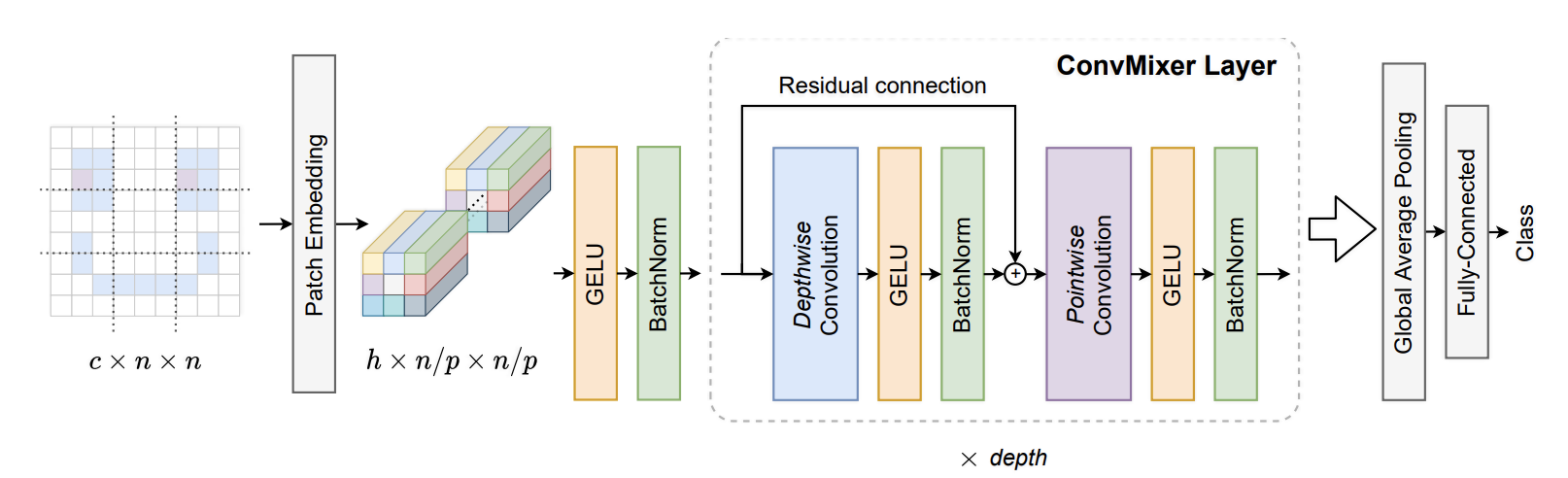# Patches Are All You Need? (ConvMixer)

This is a PyTorch implementation of the paper Patches Are All You Need?.ConvMixer is Similar to MLP-Mixer. MLP-Mixer separates mixing of spatial and channel dimensions, by applying an MLP across spatial dimension and then an MLP across the channel dimension (spatial MLP replaces the ViT attention and channel MLP is the FFN of ViT).

ConvMixer uses a convolution for channel mixing and a depth-wise convolution for spatial mixing. Since it's a convolution instead of a full MLP across the space, it mixes only the nearby batches in contrast to ViT or MLP-Mixer. Also, the MLP-mixer uses MLPs of two layers for each mixing and ConvMixer uses a single layer for each mixing.

The paper recommends removing the residual connection across the channel mixing (point-wise convolution) and having only a residual connection over the spatial mixing (depth-wise convolution). They also use Batch normalization instead of Layer normalization.

Here's an experiment that trains ConvMixer on CIFAR-10.

36import torch
37from torch import nn
38
39from labml_helpers.module import Module
40from labml_nn.utils import clone_module_list

## ConvMixer layer

This is a single ConvMixer layer. The model will have a series of these.

43class ConvMixerLayer(Module):
• d_model is the number of channels in patch embeddings,
• kernel_size is the size of the kernel of spatial convolution,
52    def __init__(self, d_model: int, kernel_size: int):
57        super().__init__()

Depth-wise convolution is separate convolution for each channel. We do this with a convolution layer with the number of groups equal to the number of channels. So that each channel is it's own group.

61        self.depth_wise_conv = nn.Conv2d(d_model, d_model,
62                                         kernel_size=kernel_size,
63                                         groups=d_model,
64                                         padding=(kernel_size - 1) // 2)

Activation after depth-wise convolution

66        self.act1 = nn.GELU()

Normalization after depth-wise convolution

68        self.norm1 = nn.BatchNorm2d(d_model)

Point-wise convolution is a convolution. i.e. a linear transformation of patch embeddings

72        self.point_wise_conv = nn.Conv2d(d_model, d_model, kernel_size=1)

Activation after point-wise convolution

74        self.act2 = nn.GELU()

Normalization after point-wise convolution

76        self.norm2 = nn.BatchNorm2d(d_model)
78    def forward(self, x: torch.Tensor):

For the residual connection around the depth-wise convolution

80        residual = x

Depth-wise convolution, activation and normalization

83        x = self.depth_wise_conv(x)
84        x = self.act1(x)
85        x = self.norm1(x)

88        x += residual

Point-wise convolution, activation and normalization

91        x = self.point_wise_conv(x)
92        x = self.act2(x)
93        x = self.norm2(x)
96        return x

## Get patch embeddings

This splits the image into patches of size and gives an embedding for each patch.

99class PatchEmbeddings(Module):
• d_model is the number of channels in patch embeddings
• patch_size is the size of the patch,
• in_channels is the number of channels in the input image (3 for rgb)
108    def __init__(self, d_model: int, patch_size: int, in_channels: int):
114        super().__init__()

We create a convolution layer with a kernel size and and stride length equal to patch size. This is equivalent to splitting the image into patches and doing a linear transformation on each patch.

119        self.conv = nn.Conv2d(in_channels, d_model, kernel_size=patch_size, stride=patch_size)

Activation function

121        self.act = nn.GELU()

Batch normalization

123        self.norm = nn.BatchNorm2d(d_model)
• x is the input image of shape [batch_size, channels, height, width]
125    def forward(self, x: torch.Tensor):

Apply convolution layer

130        x = self.conv(x)

Activation and normalization

132        x = self.act(x)
133        x = self.norm(x)
136        return x

They do average pooling (taking the mean of all patch embeddings) and a final linear transformation to predict the log-probabilities of the image classes.

139class ClassificationHead(Module):
• d_model is the number of channels in patch embeddings,
• n_classes is the number of classes in the classification task
149    def __init__(self, d_model: int, n_classes: int):
154        super().__init__()

Average Pool

156        self.pool = nn.AdaptiveAvgPool2d((1, 1))

Linear layer

158        self.linear = nn.Linear(d_model, n_classes)
160    def forward(self, x: torch.Tensor):

Average pooling

162        x = self.pool(x)

Get the embedding, x will have shape [batch_size, d_model, 1, 1]

164        x = x[:, :, 0, 0]

Linear layer

166        x = self.linear(x)
169        return x

## ConvMixer

This combines the patch embeddings block, a number of ConvMixer layers and a classification head.

172class ConvMixer(Module):
• conv_mixer_layer is a copy of a single ConvMixer layer. We make copies of it to make ConvMixer with n_layers .
• n_layers is the number of ConvMixer layers (or depth), .
• patch_emb is the patch embeddings layer.
• classification is the classification head.
179    def __init__(self, conv_mixer_layer: ConvMixerLayer, n_layers: int,
180                 patch_emb: PatchEmbeddings,
181                 classification: ClassificationHead):
189        super().__init__()

Patch embeddings

191        self.patch_emb = patch_emb

193        self.classification = classification

Make copies of the ConvMixer layer

195        self.conv_mixer_layers = clone_module_list(conv_mixer_layer, n_layers)
• x is the input image of shape [batch_size, channels, height, width]
197    def forward(self, x: torch.Tensor):

Get patch embeddings. This gives a tensor of shape [batch_size, d_model, height / patch_size, width / patch_size] .

202        x = self.patch_emb(x)

Pass through ConvMixer layers

205        for layer in self.conv_mixer_layers:
206            x = layer(x)

209        x = self.classification(x)
212        return x